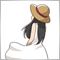# HMA indicator Of the monthly interval36

Hello Folks,

I'm new to MQL5 , I have been trying to code the HMA of the monthly timeframe but no success, I coded it to be calculated on the current timeframe then changed the interval to PERIOD_MN1 but no success

here's my code, I'd really appreciate it if someone told me what I could be doing wrong I've been stuck on it forever

```#property copyright "Aya.Adel"
#property version   "1.00"
#property indicator_chart_window
#property indicator_plots 1
#property indicator_buffers 4
#include <MovingAverages.mqh>

#property indicator_color1 Purple
#property indicator_label1 "HMA line MN"
#property indicator_type1 DRAW_LINE
//+------------------------------------------------------------------+
//| Custom indicator initialization function                         |
//+------------------------------------------------------------------+
input int HMAPeriod = 10;
input ENUM_APPLIED_PRICE HMAPrice = PRICE_CLOSE;

double HMA1stWMABuffer_MN[];
double HMA2ndWMABuffer_MN[];
double HMASumBuffer_MN[];
double HMAFinalBuffer_MN[];

int HMAHandle_MN;
int HMAHandle1_MN;

//+------------------------------------------------------------------+
//|                                                                  |
//+------------------------------------------------------------------+
int sqrt_p = (int)floor(MathSqrt(HMAPeriod));
int mid_p = (int)floor(HMAPeriod/2);

//+------------------------------------------------------------------+
//|                                                                  |
//+------------------------------------------------------------------+
int OnInit()
{
//--- indicator buffers mapping
SetIndexBuffer(0, HMAFinalBuffer_MN,INDICATOR_DATA);
SetIndexBuffer(1, HMA1stWMABuffer_MN, INDICATOR_CALCULATIONS);
SetIndexBuffer(2, HMA2ndWMABuffer_MN, INDICATOR_CALCULATIONS);
SetIndexBuffer(3, HMASumBuffer_MN, INDICATOR_CALCULATIONS);
//---
//---
HMAHandle_MN = iMA(NULL, PERIOD_MN1, mid_p, 0, MODE_LWMA, HMAPrice);
HMAHandle1_MN = iMA(NULL, PERIOD_MN1, HMAPeriod, 0, MODE_LWMA, HMAPrice);
return(INIT_SUCCEEDED);
}
//+------------------------------------------------------------------+
//| Custom indicator iteration function                              |
//+------------------------------------------------------------------+
int OnCalculate(const int rates_total,
const int prev_calculated,
const datetime &time[],
const double &open[],
const double &high[],
const double &low[],
const double &close[],
const long &tick_volume[],
const long &volume[],
{

if(rates_total<HMAPeriod)
return(0);

int to_copy;
if(prev_calculated > rates_total || prev_calculated < 0)
to_copy=rates_total;
else
{
to_copy = rates_total - prev_calculated;
if(prev_calculated> 0)
to_copy++;
}

if(IsStopped()) // checking for stop flag
return(0);
if(CopyBuffer(HMAHandle_MN, 0, 0, to_copy, HMA1stWMABuffer_MN)<=0)
{
Print("Getting 1st HMABuffer Monthly failed! Error ",GetLastError());
return(0);
}

if(IsStopped()) // checking for stop flag
return(0);
if(CopyBuffer(HMAHandle1_MN, 0, 0, to_copy, HMA2ndWMABuffer_MN)<=0)
{
Print("Getting 2nd HMABuffer Monthly failed! Error ",GetLastError());
return(0);
}

int start, iBar;
if(prev_calculated>1)
start = prev_calculated-1;
else
{
if(prev_calculated<0)
return(-1);
start=1;
}

for(iBar = start ; iBar < rates_total && !IsStopped(); iBar++)
{
HMASumBuffer_MN[iBar] = (2*HMA1stWMABuffer_MN[iBar]) - (HMA2ndWMABuffer_MN[iBar]);
}

int weightsum=0;
for(iBar = sqrt_p; iBar > 0; iBar--)
weightsum+=iBar;
//2nd buffer is a LWMA draw on the 1st HMA buffer
LinearWeightedMAOnBuffer(rates_total, prev_calculated, 0, sqrt_p, HMASumBuffer_MN, HMAFinalBuffer_MN);

//--- return value of prev_calculated for next call
return(rates_total);
}
//+------------------------------------------------------------------+
```897